### Home > PC > Chapter 8 > Lesson 8.1.4 > Problem8-61

8-61.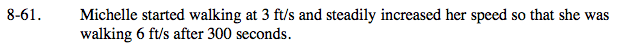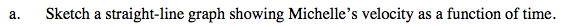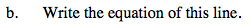Find the slope of the line and the y-intercept. Write the equation in y = mx + b form.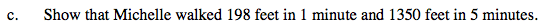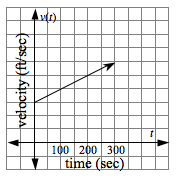Find the area under the curve after 1 minute and then after 5 minutes.

198 feet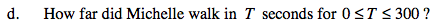$A = \frac{1}{2}(3 + (3 + 0.01T ))T = 3T + 0.005T ^{2}$